# Design of magnetic dipole based 3D integration nano-circuits for future electronics application

Document Type : Reasearch Paper

Authors

1 Department of Electronics and Telecommunication, Veer Surendra Sai University of Technology, Odisha, India.

2 Department of Electronics and Communication Engineering, Bharat Institute of Engineering and Technology, Hyderabad, India.

Abstract

Nano Magnetic Logic (NML) has been attracting application in optical computing, nanodevice formation, and low power. In this paper nanoscale architecture such as the decoder, multiplexer, and comparator are implemented on perpendicular-nano magnetic logic (pNML) technology. All these architectures with the superiority of minimum complexity and minimum delay are pointed. The proposed architectures have been designed using pNML in MagCAD tool, simulated with modelsim platform and correctness shown by simulation waveform. The correctness of these designs can be verified easily when Verilog code is generated from MagCAD tool. The performance of the proposed comparator towards default parameters shows the area of 2.4336 µm2 and critical path of 1.5E-7 sec. As a higher order, the realization of a 4-to-1 multiplexer in NML has also been included in this work.

Keywords20.1001.1.20088868.2018.9.4.5.5

Main Subjects

#### Full Text

INTRODUCTION

In this paper, we further explored digital circuit’s architectures using this tool . We designed comparator, decoder and multiplexer circuits using three-dimensional nano magnetic logic circuits. In Section 2, background related to perpendicular-nano magnetic logic circuits are described. In section 3, a new 3-D architecture of 1-bit comparator, 2to4 size decoder, 2to1 and 4to1 size multiplexer’s structures are proposed. Finally, in section 4 conclusion is present.

MATERIALS AND METHODS

Quantum-Dot Cellular Automata (QCA) is an emerging technology which has the ability to replace current CMOS technology . It has the beneficial features like higher device density, scalability, higher speed of computation, robust design, lower complexity etc. According to Physical implementation QCA technology is divided to four types (i.e. Metal-island QCA, Semiconductor QCA, Molecular QCA and Magnetic QCA). The sizes of metal-island QCA cell were demonstrated as relatively large in micrometer dimensions. The major disadvantage of this type of QCA is that its operation temperature is extremely low, which is in the range of milli-kelvin [23-24], which prevents the construction of complex QCA circuits running at room temperature. Therefore, this technology is not suitable for future electronics application. A semiconductor QCA cell is composed of four quantum dots manufactured from standard semiconductive materials . In semiconductor QCA, advanced fabrication process is possible similar to existing CMOS processes. However, current manufacturing processes cannot provide mass production for high performance and ultra-small semiconductor QCA devices. To date, most QCA device prototypes only have been demonstrated with semiconductor implementations. In molecular QCA, a basic cell is built upon only molecules. The molecules are expected to be as small as 1 nm or even smaller. Room-temperature operation of a molecular QCA cell has been experimentally confirmed . The difficulty in realizing molecular QCA is due to the high-resolution synthesis methods and positioning of molecule devices. New construction methods for molecular QCA, including self assembly on DNA rafts, are under investigation . However, it is still very difficult to fabricate molecular QCA systems with current technologies.

In magnetic QCA small magnetic dots are present also known as nano-magnets having different functionalities. These nano-magnets can be referred both as a logic element and a memory device, which are made of Co/Pt multilayer for perpendicular NML . Therefore magnetic QCA is called as nano magnetic logic. Although it’s operating frequency is low (around 100 MHz), but it has the advantage of room-temperature operation, extremely low power dissipation and high thermal robustness. A simple majority gate using this technology is already fabricated . The first large-scale QCA systems appear to be possible with a magnetic QCA circuit, which has fewer challenges during the manufacturing process compared with other implementations . Because of fabrication advantages, magnetic QCA technology is chosen in this work.

The NML technology is again split up into two types depending upon the magnetic anisotropy. Those are perpendicular NML (pNML) and in-plane NML (iNML). When the magnetic orientation of the nano magnets are in-plane; it is referred as iNML and when the orientation is perpendicular to the plane then it is referred as pNML. Both bistable logic elements logic 0 and logic 1 of iNML and pNML are shown in Fig. 1(a) and 1(b) respectively. The direction of Magnetization i.e. whether it is magnetised in one direction or the opposite, commonly referred to as “Up or Down”- is used to represent the binary information. Such as the nano magnet directed in upward direction represents a logic ‘1’ and oriented in downward direction represents a logic ‘0’.

Both pNML and iNML deal with nano magnets that interact with anti-ferromagnetic behaviour. The iNML has a complex clocking system which is eliminated in pNML . Along with it, the pNML has various advantages over iNML such as less power consumption and reduced area occupation. In addition, it also allows the fabrication on three dimensional (3-D) circuits and that too in a cost-effective way as in CMOS technology 3-D circuits comes with a huge fabrication complexity. The pNML circuits start with a nucleation centre and terminate with a pad magnet . This condition should be ensured for proper behaviour of circuit parameters.

Basic Structures of pNML

pNML is the most efficient implementation of the NML technology. In NML, the circuit complexity is reduced due to the use of minority voter (i.e. the minority gate). The minority voter and the inverters are treated as the basic functional units in pNML.

In Fig. 2(a) the pNML structure of an inverter is shown where the input magnet surrounds the output magnet. The output magnet structure [Fig. 2(b)] is called artificial nucleation centre (ANC). Basically, when odd (minimum one and maximum five) numbers of inputs are provided around it, the total structure leads to a minority voter. Similarly, there are various magnetic elements in pNML performing several functions. The structure shown in Fig. 2(c) is a normal magnet (also known as domain wall) which is the basic element of this promising technology. It is mainly used to route signals. The Fig. 2(d) shows the structure of a corner magnet which is also a normal magnet but with 90rotation. In Fig. 2(e), there is a via magnet which is used to connect to a nucleation centre situated on another plane. So by use of this, we can have one or two inter-layer outputs.

The T-connection magnet shown in Fig. 2(f) is used to split the input into two different directions. The cross-connection magnet shown in Fig. 2(g) is also a simple magnet that splits the input in three different directions. The Fig. 2(h) shows a pad magnet which is mainly used as the termination of a magnetic wire. Only a nucleation centre can be used as its output. To implement different functions in pNML we need to provide certainly fixed inputs. The structures shown in Fig. 2(i) and Fig. 2(j) are the fixed ʽ0’ and fixed ʽ1’ input magnets respectively.

A minority voter is shown in Fig. 3. Here there are three numbers of inputs namely A, B and C. All these inputs are connected to nucleation centres, nucleation centres are connected to domain walls, domain walls are connected to corner magnets and corner magnets are connected to pad-magnets. Here A, B and O outputs are in the same layer, whereas C input is in a different layer. The output expression is

Y= M(A, B, C) =. (1)

Unlike molecular QCA, if any of its inputs are fixed to 0 then it gives the NAND logic output and if the input is fixed to 1 then it behaves as a NOR gate. In the minority voter, it is necessary to have equal geometry and distance of the three inputs to the nucleation centre of the output for the proper operation of the gate. In pNML for proper propagation of the information within the circuit a perpendicular clock field is applied and after every clock cycle, the output of each building block is placed in anti-parallel compared to the previous input . Because of this reversal mechanism, the output of every building block can be used as input for next block. So signal propagation takes place in a step by step manner according to the clocking field.

RESULTS AND DISCUSSIONS

The proposed works are based on pNML technology. In pNML all the layout designs start with a nucleation centre i.e. the initial input is given to the nucleation centre. Here a 1-bit comparator, a 2to4 decoder, a 2to1 multiplexer (MUX) and a 4to1 multiplexer circuit’s layouts using pNML concept is proposed. All the layouts are designed using MagCAD tool . After the formation of layouts, VHDL netlist will be extracted. This netlist file is the replication of the circuit behaviour, which functionality is tested using ModelSim simulator, with providing proper test bench. Geometrical and physical parameters are provided in Table 1.

The comparator is a digital electronic circuit that compares two numbers. Here in this work the layout of a 1-bit comparator using the pNML technology has been proposed. In this circuit there are two 1-bit inputs i.e. A and B and it produces three outputs (1-bit) such as P for input A is less than input B (A<B), Q for A equals to B (A=B) and R for A is greater than B (A>B) which is shown in Fig. 4(a).

The minority gate schematic diagram is shown in Fig. 4(b) and pNML layout is illustrated in Fig. 4(c). The expressions for comparator outputs are derived in below equations using minority voters.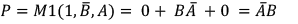(2)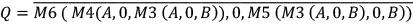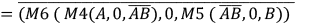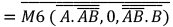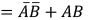(3)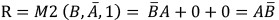(4)

The proposed design is a 3-D circuit because the magnetic wires are in different layers. According to the nano-magnetic phenomenon an input in a particular layer is propagated in its inverted form in another layer i.e. we can get the invert of an input by just changing the layer.

Here both the inputs A and B are given to the circuit layer ‘0’ initially. To get the P output, the input A is applied to the minority voter directly in layer ‘0’ but the input B is applied in layer ‘1’ so as to provide its inverted form without using an inverter circuit. Then a fixed ‘1’ input is given to the other input terminal of the minority voter. The basic concept of perpendicular-nano magnetic logic states is that if any of the inputs of a minority voter is being logic ‘0’ then it will function as a NOR gate. Hence the minority voter produces the required output P. Similarly to get the R output, the input A is applied in the same layer ‘0’ to the minority voter but input B is applied in layer ‘1’ to get the inverted B. Here also a fixed ‘1’ input is given to the minority voter and then it produces the output R which is high when input A is greater than input B. To get the output Q, it requires four numbers of minority voters along with an inverter which in term forms an Ex-NOR gate.

The above Fig. 5 shows the simulation result of the proposed layout of the 1-bit comparator. The result clearly shows that the proposed design successfully satisfies the truth table of the 1-bit comparator. The total design occupies a bounding box area of 2.4336 µm2 and it uses only six numbers of minority voters and one inverter in the total circuit. The circuit shows a critical path delay of 0.15µs. It also exhibits different latencies for different input combinations which are tabulated below.

Decoders are also called as function selector, which converts n number of inputs to 2n numbers of outputs. So its standard size is n×2n. Here a 2×4 decoder design is proposed, which is implemented using the pNML concept. The block diagram is shown in Fig. 6(a). The Fig. 6(b) and Fig. 6(c) shows the minority gate schematic and proposed pNML layout of the 2:4 decoder respectively. It is an active high output decoder, i.e. only one output bit is high at a time. In the pNML layout, four numbers of minority voters and inverters are used. Here A and B are the inputs to the decoder circuit. In the layout of all minority voters fixed 0 inputs are used which are behaving as NAND logic gates where outputs are D0=A’B’, D1=A’B, D2=AB’ and D3=AB.

The boolean expressions for the decoder outputs (Fig. 6(b)) using minority gates are shown in below equations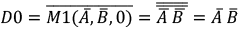(5)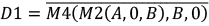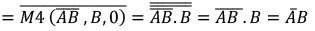(6)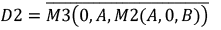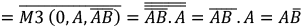(7)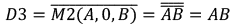(8)

The layout occupies a bounding box area of 1.0368µm2. It has a critical path delay of 0.142µs. The Fig. 7 shows the simulation result of the proposed 2to4 decoder. Here there is a latency of 870 ns for D0, 1620 ns for D1, 860 ns for Dand 850 ns for D3 outputs. The Table 3 below shows all the features of the proposed design along with the delays for several input combinations.

The multiplexer is a universal circuit, which is also known as Data Selector, Many to One, and Parallel to Serial Converter. Here a 2to1 MUX has been implemented using the perpendicular-nano magnetic logic. The block diagram and minority-gates schematic diagram are shown in Fig. 8(a) and 8(b) respectively. The pNML layout of the proposed MUX having two input lines namely Iand I1, are applied to the circuit through the nucleation centres. This is illustrated in Fig. 8(c). The 2to1 MUX design proposed in this paper comprises only three numbers of minority voters without any inverter circuit.

In pNML, logic ‘0’ in a particular layer behaves as logic ‘1’ in another layer. This property of pNML has been exploited to design the proposed layout of the MUX circuit. The selection input S is provided to the initial two minority gates in two different layers. Hence one of the two minority voters is getting a direct input S and the other is getting its inverted one i.e. S՚.

This design is based on NAND realization of 2to1 MUX. All the three minority voters in the layout are having a fixed ‘0’ input along with other two inputs; so that these minority voters behave as NAND gates. Hence the circuit finally produces an output as Y=I0S+I1S՚. The inputs are applied in different layers rather than using any additional inverting circuit which reduces the complexity of the proposed circuit layout. The Boolean expression for 2to1 MUX using minority gates is given below.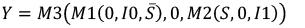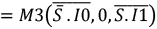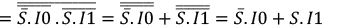(9)

This design occupies a bounding box area of 0.8056µm2. It exhibits a critical path delay of 0.14µs. The simulation result of the proposed layout of 2to1 MUX is shown in Fig. 9 below. Here from the waveform, it is shown that when the selection line S is being 0, the circuit output Y is equal to Ivalue and when S is equal to 1, then the circuit output will be Ivalue. Here Iis set as logic ‘1’ and I1 is set to logic ‘0’. For S=0 we are getting Y=1 with a latency of 855 ns and for S=1, we are getting Y=0 with a delay of 1000 ns. The same has been tabulated below in Table 4.

In this paper, a novel 4to1 size multiplexer pNML layout is also proposed which is formed by the proper connection of three numbers of 2to1 multiplexers proposed earlier in this work. As each 2to1 MUX consists of three numbers of minority voters, so a total of nine number of minority voters are used to design the whole 4to1 MUX circuit.

The block diagram and pNML layout are illustrated in Fig. 10 (a) and (b) respectively. This design is also free from inverters. Here there are four input lines i.e. I0, I1, I2 and I3 along with two numbers of selection lines Sand S1. All the inputs except I0, are in layer ‘1’ and I0 is in layer ‘0’. The layers of the inputs are changed in some cases before applying it to the minority voters in order to get its inverted form as per our requirement.

Fig. 11 shows the simulation result of the proposed layout of 4to1 MUX. From this result, we can conclude that the proposed layout completely satisfies the truth table of 4to1 MUX. The proposed circuit produces the expected output with some delay which has been tabulated below in Table 5.

Here the input values are set as I0=1, I1=0, I2=1 and I3=0. From the simulation result it can be seen that when the selection inputs S0 and S1 are being 0, the circuit produces the output Y as 1 (as I0=1) with a latency of 1144 ns. Similarly, for S1=0 and S0=1, we are getting Y=0 with a latency of 1132ns, for S1=1 and S0=0, the circuit is producing the output Y=1 and for S1=1 and S0=1, Y is being 0 with a latency of 1535ns. The total design occupies a bounding box area of 2.9376µmand exhibits a critical path delay of 0.159 µs. The below Table 6 shows the comparison of the proposed NML circuits with the existing CMOS technology. The comparative performance results in Table 6, also proven that the nano-magnetic based implementation of the comparator, decoder and multiplexer has efficient results in all the primitives as compared to the existing CMOS implementation.

CONCLUSION

In the article, we have designed nano-magnetic based computing in emerging devices as a promising domain for high speed and high-density integration. A new synthesis of implementing magnetic dipole based comparator, decoder and multiplexer are presented. These proposed architectures produce less latency which is easily verified by performance analysis tables. The latency results depict that the fast synchronization of inputs and outputs and shows an efficient implementation in the physical foreground. All the layouts proposed in this paper are implemented on the MagCAD tool using pNML technology. We have shown that the nano magnetic logic offers superior results with miniaturization of the area for proposed designs as compared to existing 45nm CMOS technology. The grid size and magnet width of all the magnetic elements are set as 120nm and 40nm respectively. MagCAD tool generates VHDL file for the designed layout and to test the functionality of the newly designed circuits. This file functionality is later verified in the ModelSim software. The miniaturisation of area properties of the nano-magnetic based present designs has afforded avenues for the miniaturizations and implementation of future electronics applications.

CONFLICT OF INTEREST

The authors declare that there are no conflicts of interest regarding the publication of this manuscript.

#### References

1.

1. Stamps R. L., Breitkreutz S., Åkerman J., Chumak A. V., Otani Y., Bauer G. E., Prejbeanu I. L., (2014), The 2014 magnetism roadmap. J. Phys. D: Appl. Phys. 47: 333001-333006.
2. Fischbacher T., Franchin M., Bordignon G., Fangohr H., (2007), A systematic approach to multiphysics extensions of finite-element-based micromagnetic simulations: Nmag. IEEE Transac. Magn. ٤٣: 2896-2898.
3. Donahue M. J., (1999), OOMMF user’s guide, version 1.0 (No. ٦٣٧٦).
4. Vansteenkiste A., Leliaert J., Dvornik M., Helsen M., Garcia-Sanchez F., Van Waeyenberge B., (2014), The design and verification of MuMax3. AIP Adv. 4: 107133-107138.
5. Riente F., Turvani G., Vacca M., Roch M. R., Zamboni M., Graziano M., (2017), Topolinano: A cad tool for nano magnetic logic. IEEE Transac. Comp. Aided Desig. Integ. Circ. Sys. ٣٦: ١٠٦١-١٠٧٤.
6. Turvani G., Riente F., Cairo F., Vacca M., Garlando U., Zamboni M., Graziano M., (2017), Efficient and reliable fault analysis methodology for nanomagnetic circuits. Int. J. Circ. Theory and Applicat. ٤٥: ٦٦٠-٦٨٠.
7. Riente F., Garlando U., Turvani G., Vacca M., Roch M. R., Graziano M., (2017), MagCAD: Tool for the Design of 3-D Magnetic Circuits. IEEE J. Explor. Solid-State Comput. Dev. Circ. ٣: ٦٥-٧٣.
8. Niemier M. T., Bernstein G. H., Csaba G., Dingler A., Hu X. S., Kurtz S., Varga E., (2011), Nanomagnet logic: Progress toward system-level integration. J. Phys.: Condens. Matter. 23: 493202-493207.
9. Niemier M., Csaba G., Dingler A., Hu X. S., Porod W., Ju X., Lugli P., (2012), Boolean and non-boolean nearest neighbor architectures for out-of-plane nanomagnet logic. In Cellular Nanoscale Networks and Their Applications (CNNA), 2012 13th International Workshop on (pp. ١-٦). IEEE.
10. Becherer M., Kiermaier J., Breitkreutz S., Eichwald I., Csaba G., Schmitt-Landsiedel D., (2013), Nanomagnetic logic clocked in the mhz regime. In Solid-State Device Research Conference (ESSDERC), 2013 Proceedings of the European (pp. ٢٧٦-٢٧٩). IEEE.
11. Becherer M., Gamm S. B. V., Eichwald I., Žiemys G., Kiermaier J., Csaba G., Schmitt-Landsiedel D., (2016), A monolithic 3D integrated nanomagnetic co-processing unit. Solid-State Elect. ١١٥: ٧٤-٨٠.
12. Riente F., Ziemys G., Turvani G., Schmitt-Landsiedel D., Gamm S. B. V., Graziano M., (2016), Towards logic-in-memory circuits using 3d-integrated nanomagnetic logic. In Rebooting Computing (ICRC), IEEE International Conference on (pp. ١-٨). IEEE.
13. Becherer M., Breitkreutz S., Eichwald I., Ziemys G., Kiermaier J., Csaba G., Schmitt-Landsiedel D., (2015), Low-power 3D integrated ferromagnetic computing. In Ultimate Integration on Silicon (EUROSOI-ULIS), 2015 Joint International EUROSOI Workshop and International Conference on (pp. ١٢١-١٢٤). IEEE.
14. Riente F., Ziemys G., Mattersdorfer C., Boche S., Turvani G., Raberg W., Breitkreutz-v. Gamm S., (2017), Controlled data storage for non-volatile memory cells embedded in nano magnetic logic. AIP Adv. ٧: ٠٥٥٩١٠-٠٥٥٩١٦.
15. Pala D., Causapruno G., Vacca M., Riente F., Turvani G., Graziano M., Zamboni M., (2015), Logic-in-memory architecture made real. In Circuits and Systems (ISCAS), 2015 IEEE International Symposium on (pp. ١٥٤٢-١٥٤٥). IEEE.
16. Cofano M., Santoro G., Vacca M., Pala D., Causapruno G., Cairo F., Zamboni M., (2015), Logic-in-memory: A nano magnet logic implementation. In VLSI (ISVLSI), 2015 IEEE Computer Society Annual Symposium on (pp. ٢٨٦-٢٩١). IEEE.
17. Cairo F., Turvani G., Riente F., Vacca M., Gamm S. B. V., Becherer M., Zamboni M., (2015), Out-of-plane NML modeling and architectural exploration. In Nanotechnology (IEEE-NANO), 2015 IEEE 15th International Conference on (pp. ١٠٣٧-١٠٤٠). IEEE.
18. Breitkreutz S., Kiermaier J., Yilmaz C., Ju X., Csaba G., Schmitt-Landsiedel D., Becherer M., (2011), Nanomagnetic logic: Compact modeling of field-coupled computing devices for system investigations. J. Comput. Elec. 10: 352-359.
19. Breitkreutz S., Kiermaier J., Eichwald I., Hildbrand C., Csaba G., Schmitt-Landsiedel D., Becherer M., (2013), Experimental demonstration of a 1-bit full adder in perpendicular nanomagnetic logic. IEEE Transact. Magnet. 49: 4464-4467.
20. Eichwald I., Breitkreutz S., Ziemys G., Csaba G., Porod W., Becherer M., (2014), Majority logic gate for 3D magnetic computing. Nanotechnol. 25: 335202-335207.
21. Žiemys G., Giebfried A., Becherer M., Eichwald I., Schmitt-Landsiedel D., Gamm S. B. V., (2016), Modeling and simulation of nanomagnetic logic with cadence virtuoso using Verilog-A. Solid-State Elec. ١٢٥: ٢٤٧-٢٥٣.
22. Misra N. K., Wairya S., Singh V. K., (2016), Optimized approach for reversible code converters using quantum dot cellular automata. In Proceedings of the 4th International Conference on Frontiers in Intelligent Computing: Theory and Applications (FICTA) 2015 (pp. 367-378). Springer, New Delhi.
23. Misra N. K., Sen B., Wairya S., Bhoi B., (2017), Testable novel parity-preserving reversible gate and low-cost quantum decoder design in 1D molecular-QCA. J. Circuits Sys. Comput. 26: 1750145-1750151.
24. Misra N. K., Wairya S., Sen B., (2017), Design of conservative, reversible sequential logic for cost efficient emerging nano circuits with enhanced testability. Ain Shams Eng. J. In Press, Corrected Proof.
25. Smith C. G., Gardelis S., Rushforth A. W., Crook R., Cooper J., Ritchie D. A., Pepper M., (2003), Realization of quantum-dot cellular automata using semiconductor quantum dots. Superlat. Microstruct. 34: 195-203.
26. Misra N. K., Sen B., Wairya S., (2016), Designing conservative reversible n-bit binary comparator for emerging quantum-dot cellular automata nano circuits. J. Nanoengin. Nanomanuf. 6: 201-216.
27. Hu W., Sarveswaran K., Lieberman M., Bernstein G. H., (2005), High-resolution electron beam lithography and DNA nano-patterning for molecular QCA. IEEE Transac. Nanotechnol. ٤: ٣١٢-٣١٦.
28. Zeper W. B., Van Kesteren H. W., Jacobs B. A. J., Spruit J. H. M., Carcia P. F., (1991), Hysteresis, microstructure, and magneto‐optical recording in Co/Pt and Co/Pd multilayers. J. Appl. Phys. ٧٠: ٢٢٦٤-٢٢٧١.
29. Imre A., Csaba G., Ji L., Orlov A., Bernstein G. H., Porod W., (2006), Majority logic gate for magnetic quantum-dot cellular automata. Science. ٣١١: ٢٠٥-٢٠٨.
30. Sharma A., Singh R., Kajla P., (2013), Area efficient 1-bit comparator design by using hybridized full adder module based on PTL and GDI logic. Int. J. Comp. Applic. ٨٢: ٥-١٣.
31. Rahi P. K., Dewangan S., Bhagel S., (2015), Design and Simulation of 2–TO-4Decoder using 32nm, 45nm AND 65nm CMOS Technology. Int. J. Sci. Res. Eng. Technol. (IJSRET). ٤: ٢٧٠-٢٧٣.
32. Chien J. C., Lu L. H., (2006), A 15-gb/s 2 : 1 multiplexer in 0.18-/spl mu/m CMOS. IEEE Micro. Wireless Comp. Lett. 16: 558-560.